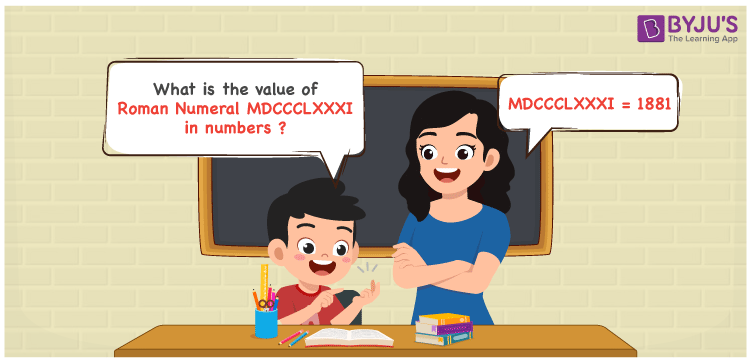Checkout JEE MAINS 2022 Question Paper Analysis : Checkout JEE MAINS 2022 Question Paper Analysis :

# MDCCCLXXXI Roman Numerals

MDCCCLXXXI Roman Numerals is 1881. This article helps you learn the method of writing MDCCCLXXXI as 1881 in numbers in an easy to understand way. The roman numerals conversion articles are designed in a simple language to help students recall the procedure without any help. Therefore, the roman numerals MDCCCLXXXI is written as 1881 in numbers.

 Number Roman Numeral 1881 MDCCCLXXXI## How to Write MDCCCLXXXI Roman Numerals in Numbers?

This section offers a great knowledge of the roman numerals expansion and conversion to numbers.

MDCCCLXXXI = M + D + C + C + C + L + X + X + X + I

MDCCCLXXXI = 1000 + 500 + 100 + 100 + 100 + 50 + 10 + 10 + 10 + 1

MDCCCLXXXI = 1881

## Video Lesson on Roman Numerals## Frequently Asked Questions on MDCCCLXXXI Roman Numerals

### Why is MDCCCLXXXI the roman numeral of 1881?

We know that

M is the roman numerals of 1000

DCCC is the roman numerals of 800

LXXX is the roman numerals of 80

I is the roman numerals of 1

So MDCCCLXXXI is the roman numeral of 1881.

### Determine 1900 – 19.

We know that

1900 – 19 = 1881

Hence, 1900 – 19 is 1881 which is written as MDCCCLXXXI.

### Find the remainder if MDCCCLXXXI is divided by XVII.

We know that

MDCCCLXXXI = 1881

XVII = 17

The remainder if MDCCCLXXXI is divided by XVII is XI.